Soccer team

Hilahs soccer team is trying to raise \$2414 to travel to a tournament in Florida, so they decided to host a pancake for the breakfast. How many people need to attend their breakfast in order to raise \$2414, if profit per one pancake is \$1.5?

Result

n =  1608

Solution:Leave us a comment of this math problem and its solution (i.e. if it is still somewhat unclear...):Be the first to comment!Next similar math problems:

1. Shirt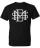Mrs. Vítková bought each of his three children the same shirt paid CZK 1,000. Saleswoman she returned 568,60 CZK. What was the price of one shirt?
2. CustomerA customer purchase three (3) writing pads from a store. She receive k9.70 change from a k10.00 note . How much was it's writing pad cost?
3. Soda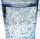A pack of 12 sodas cost 5.04. What is the unit rate in dollars per soda?
4. Cheaper cars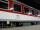State-owned railway company buys 10 air-conditioned coaches for nearly 18 million euros. Calculate how many euros is the equivalent of one seat in the wagon with a capacity of 83 people. How many of used cars at the price 2700 Euros can be buy instead o
5. PercentagesWhat is 1/3 of \$821.43?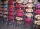Cris had 15000 . He spent 1/3 of his money on a table and the remaining on 5 similar chairs that cost the same. How much did one chair cost?
7. Far country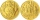In a country far away, the value of 3 pesos is 12 centavos more than the value of 1 peso. How many centavos is 1 peso worth?
8. Money 6Lita had some money. She spent 1/2 of it on a hand bag and 1/3 of the remainder of the blouse. She had 260.00. How much money did she have at start?
9. VAT lottery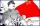Father earns 993 euro a month, mother 918 euro per month. Calculate what amount they paid as value added tax (VAT) to the government if the VAT rate is 20%. Assume that the family will spend their entire monthly income.
10. DisjointHow many elements have union and intersection of two disjoint sets when the first have 1 and secodn 8 elements.
11. Unknown number 11That number increased by three equals three times itself?
12. Passenger boatTwo-fifths of the passengers in the passenger boat were boys. 1/3 of them were girls and the rest were adult. If there were 60 passengers in the boat, how many more boys than adult were there?
13. Round it0.728 round to units, tenths, hundredths.
14. DecideThe rectangle is divided into seven fields. On each box is to write just one of the numbers 1, 2 and 3. Mirek argue that it can be done so that the sum of the two numbers written next to each other was always different. Zuzana (Susan) instead argue that.
15. AlleyAlley measured a meters. At the beginning and end are planted poplar. How many we must plant poplars to get the distance between the poplars 15 meters?
16. Equation 29Solve next equation: 2 ( 2x + 3 ) = 8 ( 1 - x) -5 ( x -2 )
17. Simple equation 9Solve the following equation: -8y+5=-9y+9